## 1: Introduction to the algorithm

Early estimation of ADMET reduces drastically the fraction of pharmacokinetics-related failure in later development stages.

A series of web-based AI models that allow absorption, distribution, metabolism, excretion and toxicity assessment for small molecules have been developed. These models allow users to compute physicochemical descriptors，as well as to predict pharmacokinetic properties, and to evaluate drug-like nature of one or multiple small molecules.

## 2: Start to Use

iDrug provides two methods of inputting data, including inputting SMILES, and uploading molecular file.

• Input SMILES

Select the "Input your SMILES" check box, then enter one or more SMILES in the text box. Multiple SMILES should be separated by commas (,).

You can also click the "DRAW" icon at the end of the text box to pop up the molecular editor, in which you can draw the molecular structure (only one) and click "Confirm" to convert it to SMILES which will be displayed in the text box.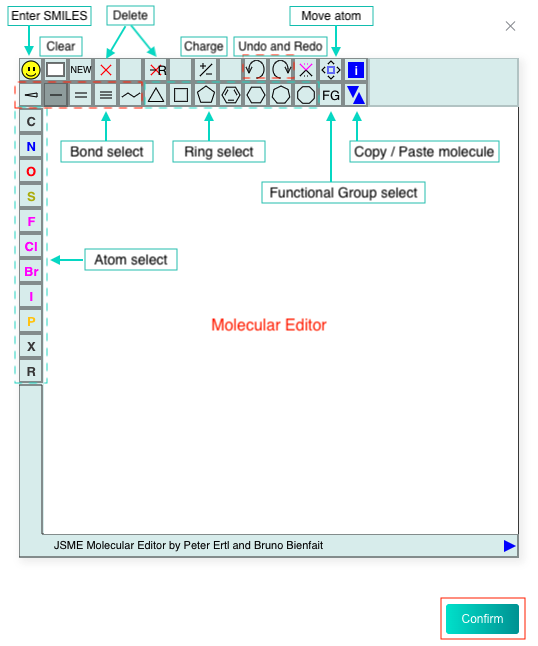Select the "Upload SDF file with 3D conformation" check box, then click the "Click to upload" button to upload a file.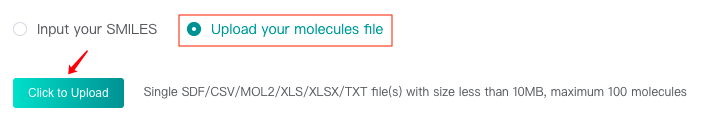Your file you needs to meet these requirements:

• The format should be sdf/csv/mol2/xls/xlsx/txt.
• The number of molecules in the file should not exceed 100.
• The file size should not exceed 10MB.
• The column name of SMILES should be "smiles" or "SMILES". The following figure provides an example: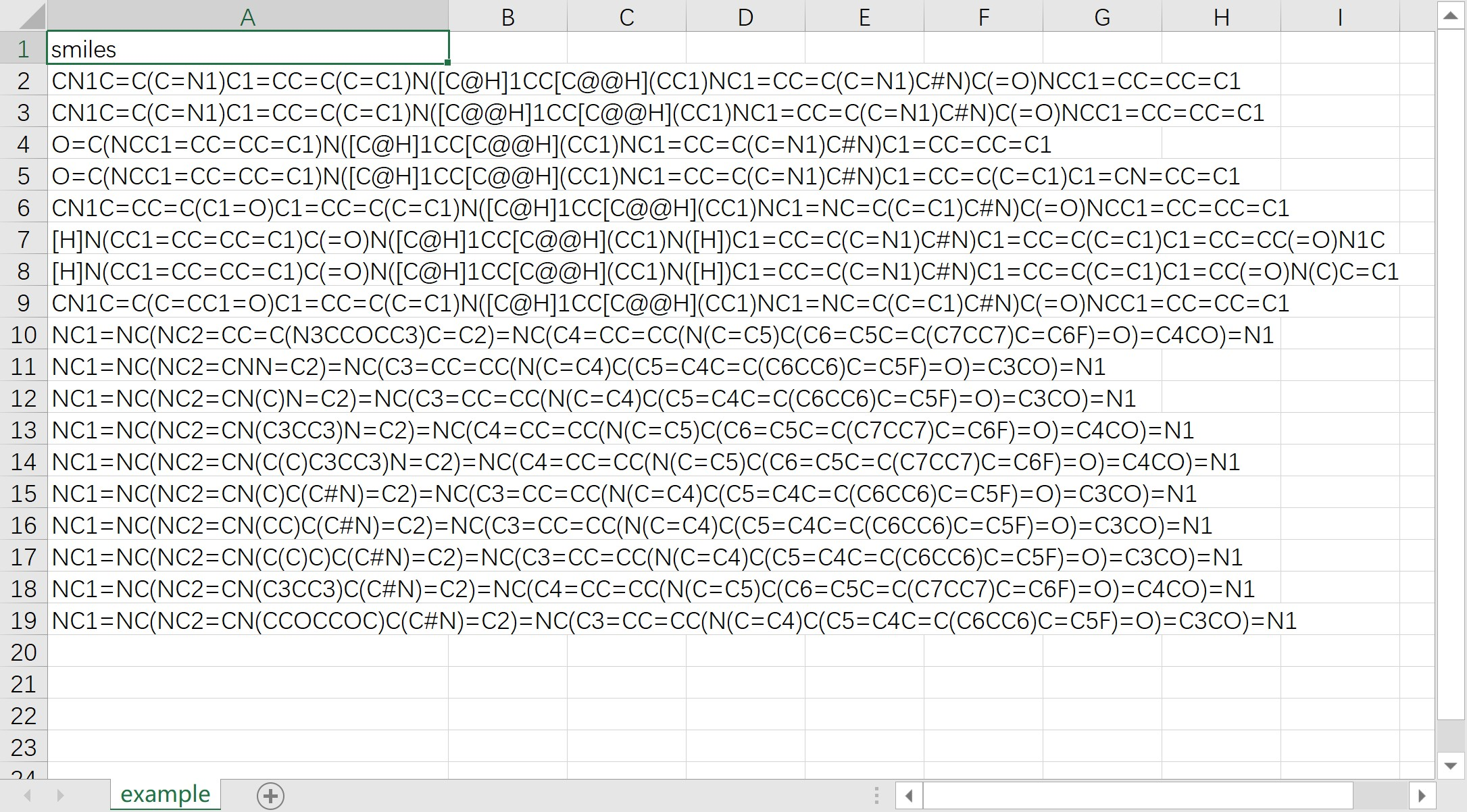After data input, click the "Submit" button to submit the task. The task will be completed within 10min and the results will be displayed.

In order to reasonably allocate limited resources, we limit the user's task quota. Hover over the "Submit" button to see the restrictions and usage. (In this module number of tasks = number of predicted molecules)#### 3.1 Query results

After the task is completed, you will get the prediction result for the input molecules.

• If you input SMILES in the text box when you input data, the output contains molecular structure diagrams, molecular radar map, and molecular properties:

1) Molecular Structure Diagrams and Molecular Radar Map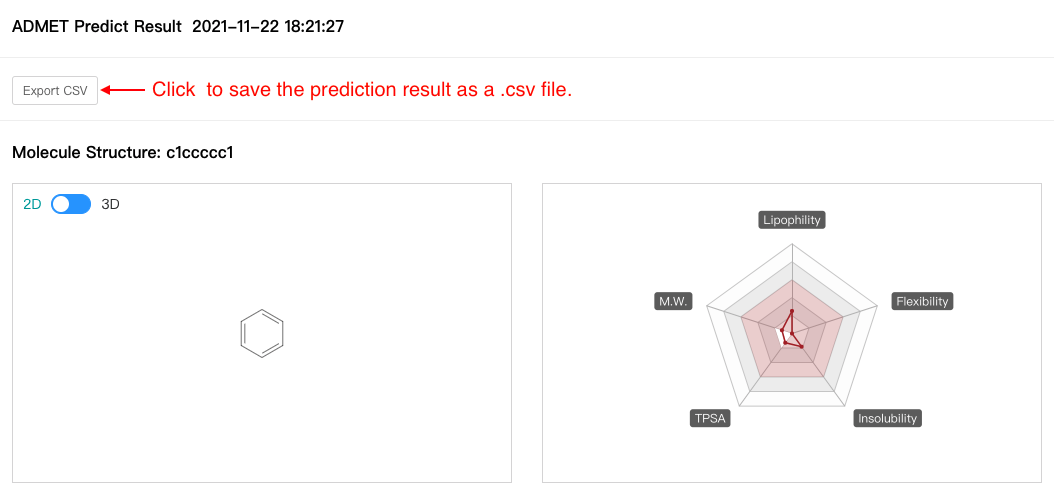2) Molecular Properties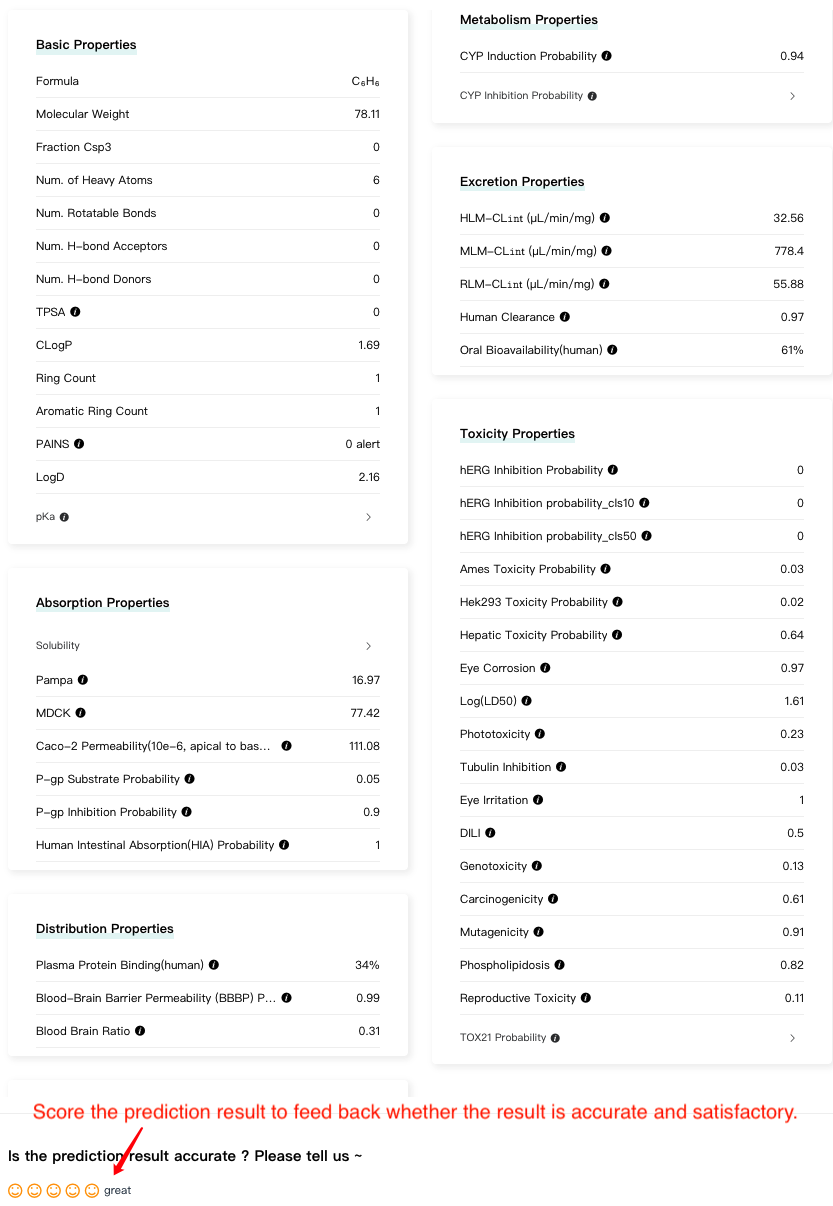• If you upload molecular file when you input data, the prediction result will be presented in a list as shown in the figure below: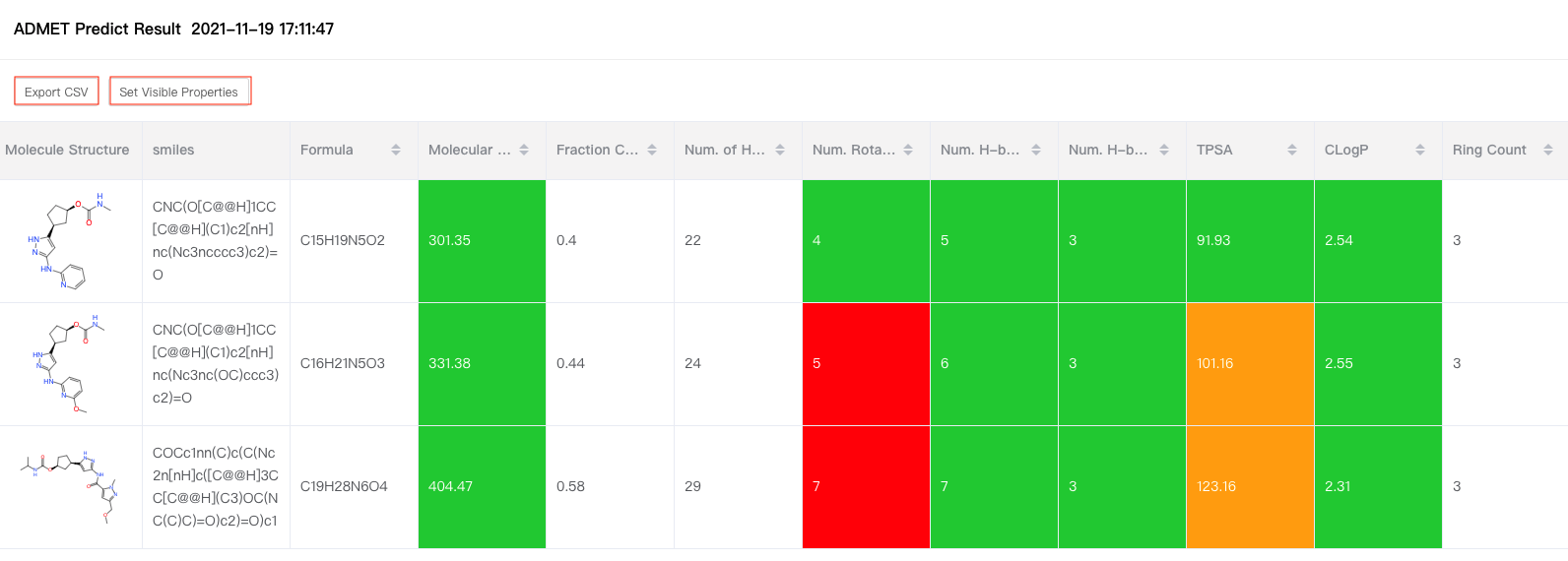#### 3.2 Query history

Click the "History" tab at the top of the page to view the results of the last 20 tasks.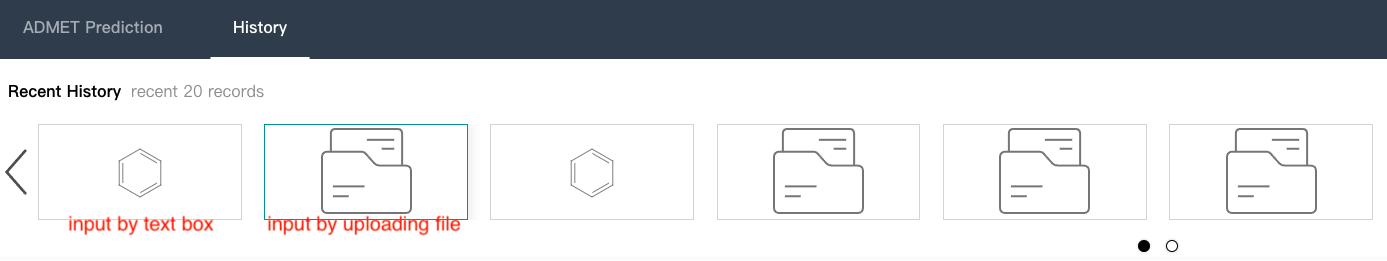Thermodynamic Solubility (abbr. ts, tsc1, tsc10, tsc100) a thermodynamic water solubility value (logrithm of mol/L) per molecule. The value is a direct measure of the solubility of the molecule. We provided four thermodynamic solubility model, where ts is a regression model, tsc1 is a classification model which cutoff is 1uM/L. Likewise, tsc10 and tsc100 use 10 uM/L and 100 uM/L as cutoff.
Caco-2 permeability (apical to basolateral) A CACO-2 permeability value (Papp，10^-6 cm/s). The value can be used to evaluate the human intestinal permeability.
Blood-Brain Barrier Permeability (BBBP) probability A probability value (between 0 and 1) per molecule. The molecule is highly possible to pass through the blood brain barrier if the value is high.
Human intestinal absorption (HIA) probability A probability value (between 0 and 1) per molecule. The molecule is highly possible to be absorbed if the value is close to 1.
Plasma Protein Binding (PPB) (human) The percentage of chemicals bound to plasma protein (between 0 and 1) per molecule.
pKa Strongest acidic pKa and strongest basic pKa per molecule. If no acidic functional group or basic functional group is found in the molecule, that line will be n/a.
Tox21 probability Qualitative toxicity measurements on 12 biological targets, including nuclear receptors and stress response pathways. Output as 12 probability value (between 0 and 1) per molecule. Each subtitle displayed on the result page implies the specific tox endpoint.
hERG inhibition probability A probability value (between 0 and 1) per molecule. The molecule is highly possible to be hERG inhibitor.
hERG inhibition probability (c10) A probability value of molecule’s IC50 value below that threshold (10 uM). If the value is close to 1, the IC50 is more likely to be lower than the threshold. Which means it is an inhibitor.
hERG inhibition probability (c50) A probability value of molecule’s IC50 value below that threshold (50 uM). If the value is close to 1, the IC50 is more likely to be lower than the threshold. Which means it is an inhibitor.
P-gp substrate probability A probability value (between 0 and 1) per molecule. The molecule is highly possible to be a p-glycoprotein substrate if the value is close to 1.
P-gp inhibition probability A probability value (between 0 and 1) per molecule. The molecule is highly possible to be a p-glycoprotein inhibitor if the value is close to 1.
logD (pH7.4) Distribution coefficients at pH 7.4 (logD7.4).
HLM-CL𝚒𝚗𝗍 (μL/min/mg) An estimate value of in vitro human liver microsome metabolic stability (base 10 logrithm of mL/min/g).
MLM-CL𝚒𝚗𝗍 (μL/min/mg) An estimate value of in vitro mouse liver microsome metabolic stability (base 10 logrithm of mL/min/g).
RLM-CL𝚒𝚗𝗍 (μL/min/mg) An estimate value of in vitro rat liver microsome metabolic stability (base 10 logrithm of mL/min/g).
CYP inhibition probability A probability value(between 0 and 1) of a molecule to be the inhibitor of CYP 1A2, 2C19, 2C9, 2D6, 3A4.
Oral Bioavailability (human) A bioavailability value (between 0 and 1) per molecule. The molecule has high oral bioavailability if the value is close to 1.
Ames Toxicity probability A probability value (between 0 and 1) per molecule. The molecule is highly possible to induce ames toxicity if the value is close to 1.
Hepatic Toxicity probability A probability value (between 0 and 1) per molecule. The molecule is highly possible to induce hepatotoxicity if the value is close to 1.
Hek293 Toxicity probability A probability value (between 0 and 1) per molecule. The molecule is highly possible to induce hek293 toxicity if the value is close to 1.
Kinetic Solubility A kinetic solubility value (base 10 logrithm of mol/L) per molecule. The value is measured at pH 7.4 and 25℃ in PBS.
PAMPA A PAMPA permeability value (Papp，10^-6 cm/s).
MDCK In vitro passive membrane permeability across multidrug resistance Madin-Darby canine kidney (MDCK) cell monolayers with unit of log(10^-6 cm/s)，the direction was apical to basal.
DILI A probability value (between 0 and 1) ). A molecule is highly possible to induce liver injury if the vale is close to 1.
Carcinogenicity A probability value (between 0 and 1) ). A molecule is highly possible to be carcinogenicity if the vale is close to 1.
Tubulin inhibition A probability value (between 0 and 1) ). A molecule is highly possible to inhibit tubulin if the vale is close to 1.
Mutageniciy A probability value (between 0 and 1) ). A molecule is highly possible to be mutagenicity if the vale is close to 1.
CYP induction A probability value (between 0 and 1) ). A molecule is highly possible to induce cytochrome P450 by evaluating mRNA levels and/or catalytic activity if the vale is close to 1.
Genotoxicity A probability value (between 0 and 1) ). A molecule is highly possible to be genotoxic if the vale is close to 1.
Reproductive toxicity A probability value (between 0 and 1) ). A molecule is highly possible to have a reproductive toxicity if the vale is close to 1.
Eye irritation A probability value (between 0 and 1) ). A molecule is highly possible to cause dryness, itching, pain, or grittiness of the eye if the vale is close to 1.
Eye corrosion A probability value (between 0 and 1) ). A molecule is highly possible to cause tissue damage in the eye, or serious physical decay of the vision if the vale is close to 1.
Phototoxicity A probability value (between 0 and 1) ). A molecule is highly possible to make the skin or eyes become very sensitive to sunlight if the value is close to 1.
phospholipidosis A probability value (between 0 and 1) ). A molecule is highly possible to induce intracellular accumulation of phospholipids in tissues if the value is close to 1.
Human clearance A human clearance value (log10 of ml/min/kg). Human clearance is a measure of excretion ability of a molecule by a human body.
Blood brain ratio A value (log10) measure the ratio of brain concentration to blood concentration of a molecule.
LD50 A median lethal dose (log10 of mg/kg). LD50 value of a molecule is the dose required to kill half the members of the tested population.